# Square facts for kids

Kids Encyclopedia Facts
(Redirected from Square (geometry))

A square is a shape with four equal sides and four corners that are all right angles (90 degrees). The diagonals of a square also cross at right angles. The angle between any diagonal and a side of a square is 45 degrees. A square has rotational symmetry of four. It has four lines of regular symmetry. A square with vertices A, B, C and D can be written as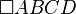$\square ABCD$.

A square is a type of rectangle with all sides of equal length. However, while a square is a type of rectangle and rhombus, the opposite does not need to be true.

A square is also the 2-dimensional analogue of a cube.

## Formulas

If the length of side a is known, then we have that:

 Perimeter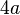$4a$ Area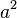$a^2$ Length of diagonal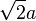$\sqrt{2}a$

If the length of diagonal d is known, then we have that:

 Length of side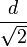$\frac{d}{\sqrt{2}}$ Perimeter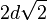$2d\sqrt{2}$ Area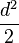$\frac{d^2}{2}$

## Images for kidsSquare Facts for Kids. Kiddle Encyclopedia.